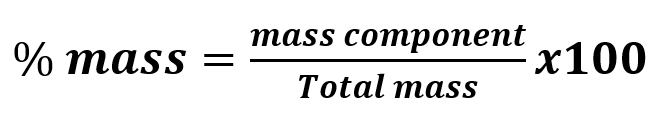# Problem: Copper can be drawn into thin wires. How many meters of 34-gauge wire (diameter = 6.304 x 10−3 in) can be produced from the copper in 5.01 lb of covellite, an ore of copper that is 66% copper by mass? (Hint: Treat the wire as a cylinder: V of cylinder = πr2h; d of copper = 8.95 g/cm3.)

###### FREE Expert Solution

First, we have to notice that the mass given was for an impure substance containing copper. This means that we have to use mass % to calculate the mass of copper.Apply the formula to the problem then rearrange to isolate mass of Cu (Copper)

91% (456 ratings)###### Problem Details

Copper can be drawn into thin wires. How many meters of 34-gauge wire (diameter = 6.304 x 10−3 in) can be produced from the copper in 5.01 lb of covellite, an ore of copper that is 66% copper by mass? (Hint: Treat the wire as a cylinder: V of cylinder = πr2h; d of copper = 8.95 g/cm3.)

Frequently Asked Questions

What scientific concept do you need to know in order to solve this problem?

Our tutors have indicated that to solve this problem you will need to apply the Density of Geometric Objects concept. You can view video lessons to learn Density of Geometric Objects. Or if you need more Density of Geometric Objects practice, you can also practice Density of Geometric Objects practice problems.

What professor is this problem relevant for?

Based on our data, we think this problem is relevant for Professor Mandziuk's class at NYU.

What textbook is this problem found in?

Our data indicates that this problem or a close variation was asked in Chemistry: The Molecular Nature of Matter and Change - Silberberg 8th Edition. You can also practice Chemistry: The Molecular Nature of Matter and Change - Silberberg 8th Edition practice problems.